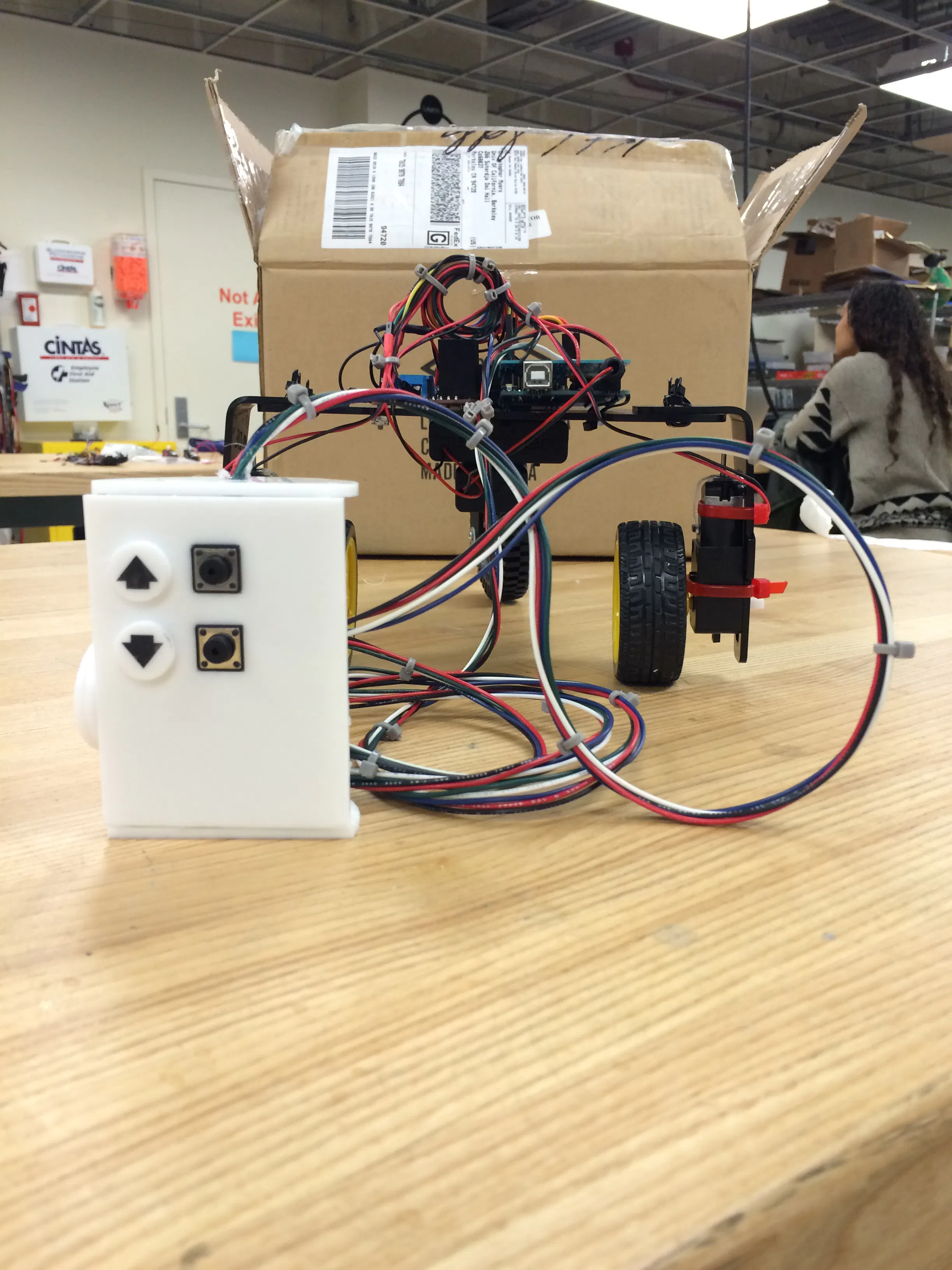Created November 14, 2015 © LGPL

# Part 3: Finished Concept Vehicle

The finished concept vehicle with a remote control!## Code

### Code using a Wired Controller

C/C++
```#include <Servo.h>

Servo myservo;  // create servo object to control a servo
// twelve servo objects can be created on most boards

int pos = 90;    // variable to store the servo position
int potpin = 2;  // analog pin used to connect the potentiometer
int val;    // variable to read the value from the analog pin

const int buttonPin = 3;     // the number of the pushbutton pin1
const int buttonPin2 = 11;
int goForward = 0;
int goBackward = 0;

// connect motor controller pins to Arduino digital pins
// motor one
int enA = 5;
int in1 = 10;
int in2 = 7;
// motor two
int enB = 6;
int in3 = 8;
int in4 = 9;

void setup()
{
pinMode(buttonPin, INPUT);
pinMode(buttonPin2, INPUT);
// set all the motor control pins to outputs
pinMode(enA, OUTPUT);
pinMode(enB, OUTPUT);
pinMode(in1, OUTPUT);
pinMode(in2, OUTPUT);
pinMode(in3, OUTPUT);
pinMode(in4, OUTPUT);
myservo.attach(A0);  // attaches the servo on pin 9 to the servo object
delay(2000);
}

void forward() {
digitalWrite(in1, HIGH);
digitalWrite(in2, LOW);
digitalWrite(in3, HIGH);
digitalWrite(in4, LOW);
}

void backward() {
digitalWrite(in1, LOW);
digitalWrite(in2, HIGH);
digitalWrite(in3, LOW);
digitalWrite(in4, HIGH);
}

void motorsOn() {
analogWrite(enB, 255);
analogWrite(enA, 255);
}

void motorsOff() {
digitalWrite(enA, 0);
digitalWrite(enB, 0);
digitalWrite(in1, LOW);
digitalWrite(in2, LOW);
digitalWrite(in3, LOW);
digitalWrite(in4, LOW);
}

void steer() {
val = analogRead(potpin);            // reads the value of the potentiometer (value between 0 and 1023)
val = map(val, 0, 1023, 90, 180);     // scale it to use it with the servo (value between 0 and 180)
myservo.write(val);                  // sets the servo position according to the scaled value
delay(40);                           // waits for the servo to get there
}

void loop() {

while (goForward == HIGH) {
forward();
motorsOn();
//    steer();
}
motorsOff();
steer();

while (goBackward == HIGH) {
backward();
motorsOn();
steer();